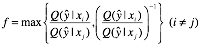# Objective is a function of individual entries of a right stochastic matrix

As stated in the title. How to do convex optimization when my variable is an n by k right stochastic matrix (k < n), whereas my objective function is a function in terms of the matrix’s individual entries?

More specifically, my matrix looks like this:And the objective function is actually:Of course, I’ll have to write something like:I just don’t know how to characterize the objective function f in terms of the matrix variable Q. It is already known that right stochastic matrices form a convex set, however, in this case, is the objective function still a convex function that I can minimize? If so, how to do it?

Thanks for any help!

If you do not know if this model is convex—and to be honest, I do not know either, but I doubt it—then you should not be attempting to use CVX, and there are much better forums to discuss the problem.

Your constraints constrain the matrix to be right stochastic, and of course are linear, so you are right that the set of right stochastic matrices is convex. But that leaves your objective function to worry about. It doesn’t look very convex to me, but I have to caveat that by not even knowing what it is defined to be, since I don’t see any subscripts for the y_hat entries in f, so don’t know how the objective function is defined in terms of your overall variable matrix.

If you are trying to minimize the maximum of any ratio of different elements in a column and its reciprocal, then, in the absence of other constraints, wouldn’t the optimum objective value be 1, achieved with all matrix elements equal to 1/n ? Anyhow, I suppose that is not really your objective function, but don’t know what is.